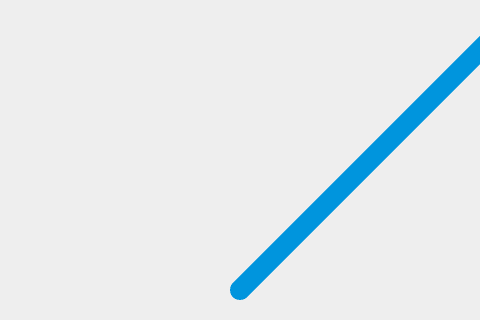# 让球动起来

``````function draw() {
// drawing code
}
setInterval(draw, 10);
``````

``````ctx.beginPath();
ctx.arc(50, 50, 10, 0, Math.PI*2);
ctx.fillStyle = "#0095DD";
ctx.fill();
ctx.closePath();
``````

## 让球动起来

``````var x = canvas.width/2;
var y = canvas.height-30;
``````

``````function draw() {
ctx.beginPath();
ctx.arc(x, y, 10, 0, Math.PI*2);
ctx.fillStyle = "#0095DD";
ctx.fill();
ctx.closePath();
}
``````

``````var dx = 2;
var dy = -2;
``````

``````function draw() {
ctx.beginPath();
ctx.arc(x, y, 10, 0, Math.PI*2);
ctx.fillStyle = "#0095DD";
ctx.fill();
ctx.closePath();
x += dx;
y += dy;
}
``````## 在每一帧更新之前清空画布

``````function draw() {
ctx.clearRect(0, 0, canvas.width, canvas.height);
ctx.beginPath();
ctx.arc(x, y, 10, 0, Math.PI*2);
ctx.fillStyle = "#0095DD";
ctx.fill();
ctx.closePath();
x += dx;
y += dy;
}
``````

## 保持代码整洁

``````function drawBall() {
ctx.beginPath();
ctx.arc(x, y, 10, 0, Math.PI*2);
ctx.fillStyle = "#0095DD";
ctx.fill();
ctx.closePath();
}

function draw() {
ctx.clearRect(0, 0, canvas.width, canvas.height);
drawBall();
x += dx;
y += dy;
}
``````